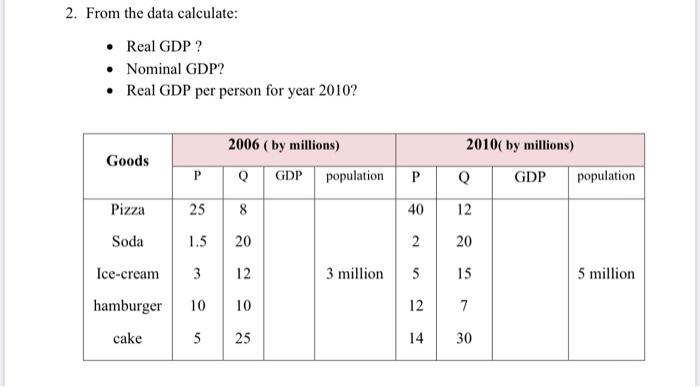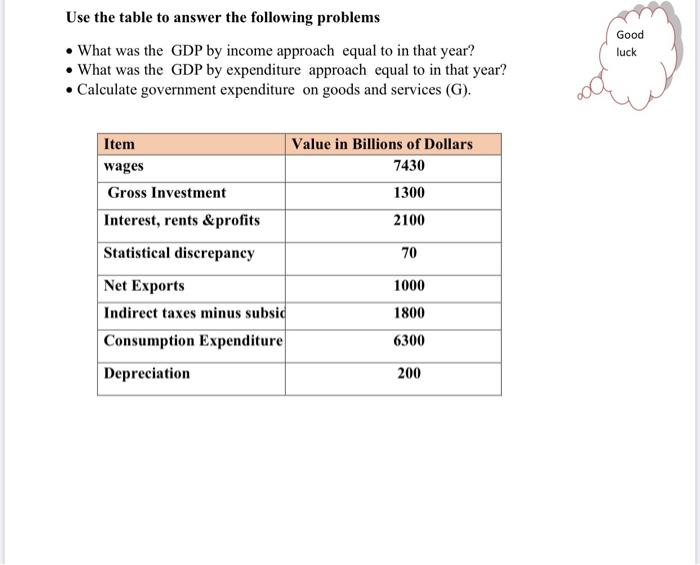### Create an Account

Home / Questions / From the data calculate: • Real GDP ? • Nominal GDP? • Real GDP per person for year 2010?...

# From the data calculate: • Real GDP ? • Nominal GDP? • Real GDP per person for year 2010? 2006 (by millions) Goods 2010( by millions) Q GDP population P Q GDP population P Pizza 25 8 40 12 Soda

From the data calculate: • Real GDP ? • Nominal GDP? • Real GDP per person for year 2010? 2006 (by millions) Goods 2010( by millions) Q GDP population P Q GDP population P Pizza 25 8 40 12 Soda 1.5 20 2 20 Ice-cream 3 12 3 million 5 15 5 million hamburger 10 10 12 7 cake 5 25 14 30
Use the table to answer the following problems What was the GDP by income approach equal to in that year? • What was the GDP by expenditure approach equal to in that year? • Calculate government expenditure on goods and services (G). Good luck Item Value in Billions of Dollars wages 7430 Gross Investment 1300 Interest, rents &profits 2100 Statistical discrepancy 70 Net Exports 1000 Indirect taxes minus subsid 1800 Consumption Expenditure 6300 Depreciation 200Apr 26 2021 View more View LessSubscribe To Get Solution